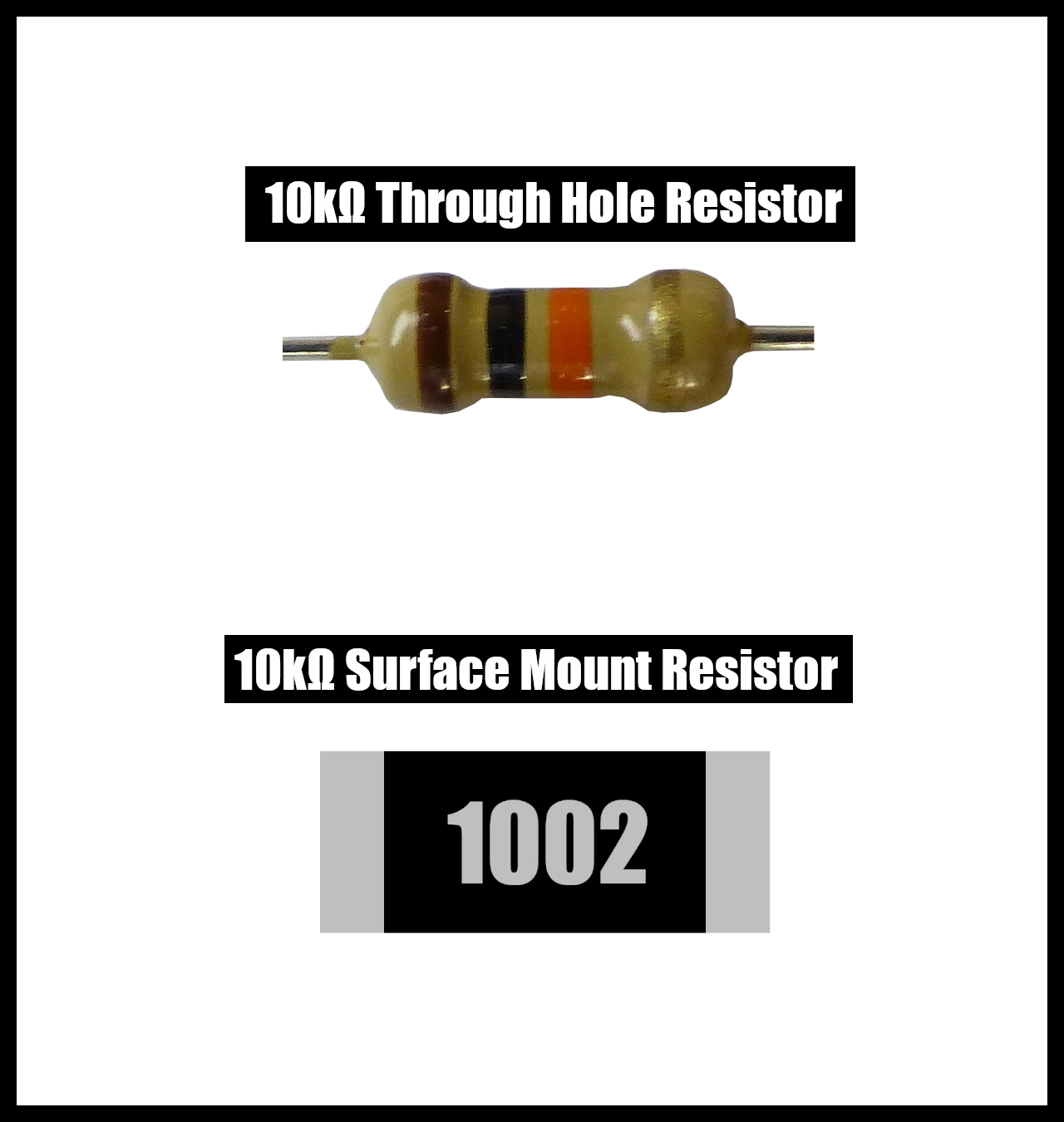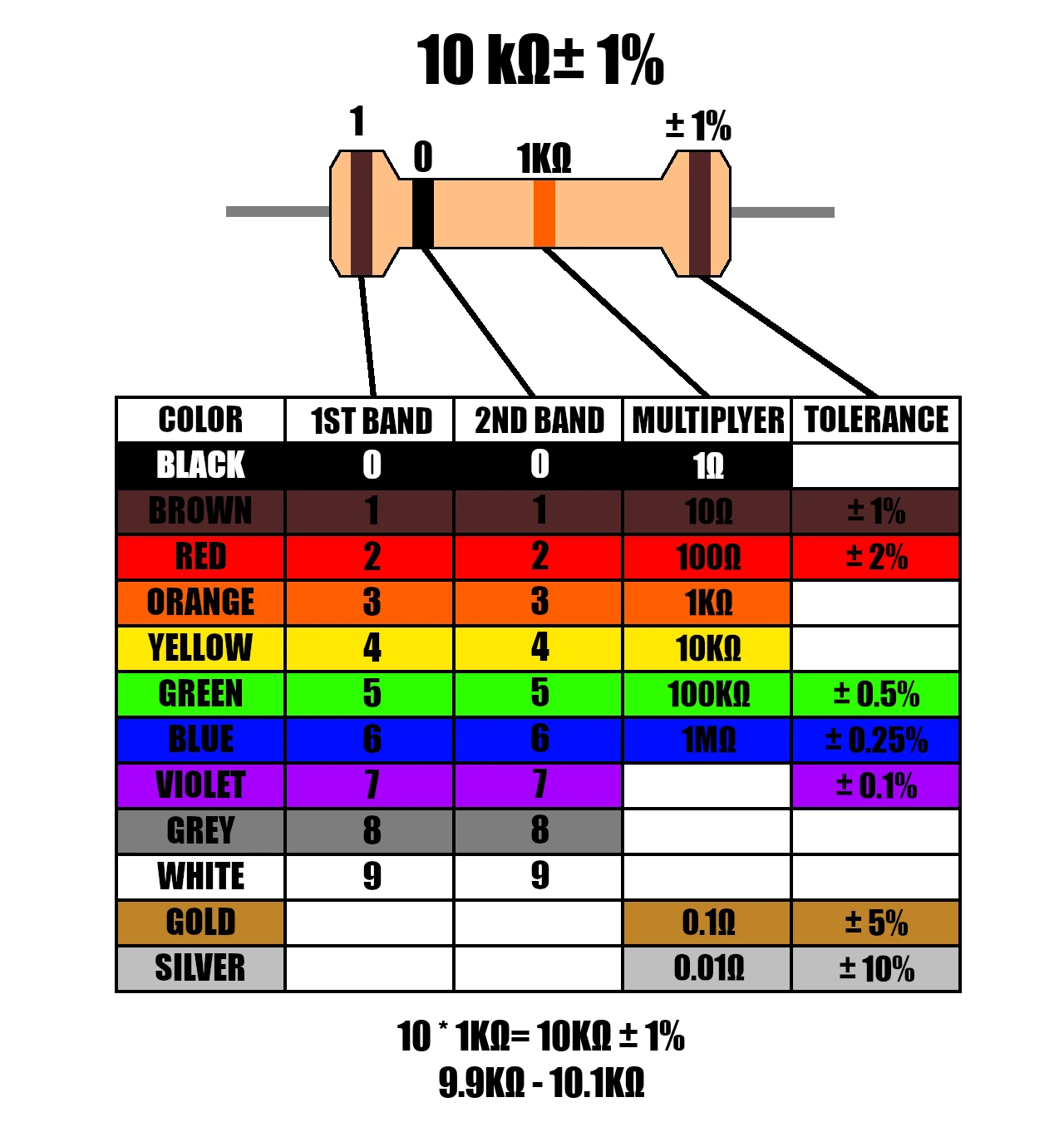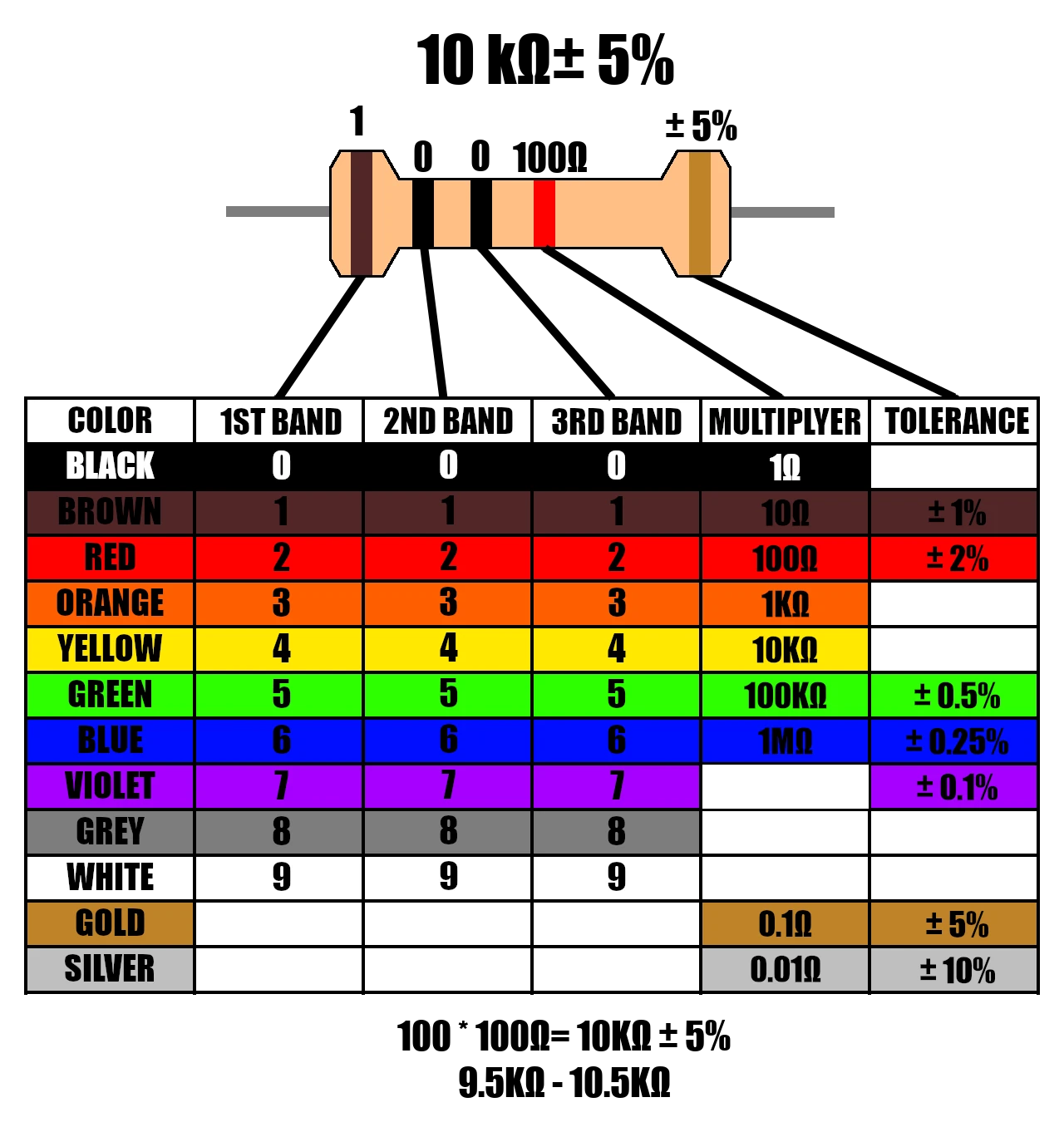Home The Quantizer> Electronics> How to identify a 10k resistor (With Images)

# How to identify a 10k resistor (With Images)# Through Hole Resistor

## Identify 10kΩ Resistor (4 bands)

The easiest way to identify a through hole 10k resistor is by looking at the color bands on it. Looking at the chart below you can see that the 1st band plus the 2nd band times the 3rd band will tell you what the value of the resistor is with a 4th band indicating the tolerance. For a 10k resistor this can be represented as

• 1st band = brown 1 (1st digit)
• 2nd band = black => 0 (2nd digit)
• 3d band = orange => 1kΩ (multiplier)
• 4th band = brown for a ±1% tolerance.
• $(10 * 1kΩ) ±1% = 10kΩ ±1%$

## Color Bands## Identify 10kΩ Resistor (5 bands)

The 5 band through hole resistor is just like the 4 band version except there is a 3rd digit indicated by the 3rd band allowing for more precision. Looking at the chart below you can see that the 1st band, 2nd band, and 3rd band are used to indicate the resistor value multiplied by the 4th band value and lastly using the 5th band to indicating the tolerance. For a 10k resistor this can be represented as

• 1st band = brown 1 (1st digit)
• 2nd band = black => 0 (2nd digit)
• 3d band = black => 0 (3nd digit)
• 4th band = red => 1kΩ (multiplier)
• 5th band = gold for a ±5% tolerance.
• $(10 * 1kΩ) ±1% = 10kΩ ±1%$

## Color Bands## Types of through hole resistors

• Carbon Composition Resistors
• Carbon Film Resistors
• Ceramic Composition Resistors
• Current Sense Resistors
• Metal Film Resistors
• Metal Foil Resistors
• Metal Oxide Resistors
• Thick Film Resistors
• Thin Film Resistors
• Wire wound Resistors

# Surface Mount

If the resistor is instead a surface mount package (most common in newer pcb circuits) than you can read the code printed on the device to see if it is a 10k resistor.

## 3 Digit EIA

A common format for SMD resistors where digit one and digit two are multiplied by the number of zeros indicated by the 3rd digit.

• 103 = 1st digit
• 103 = 2nd digit
• 103 = number of zeros to append
Ex: 103 would be $10 * 1,000 = 10,000Ω => \boxed{10kΩ}$

For resistances less than 10Ω an R is used in the 2nd digit
Ex: 1R5 = 1.5Ω resistor

## 4 Digit EIA

Similar to the 3 digit version except there is an additional digit of precision.

• 1002 = 1st digit
• 1002 = 2nd digit
• 1002 = 3rd digit
• 1002= number of zeros to append
Ex: 1002 would be $100 * 100 = 10,000Ω => \boxed{10kΩ}$

For resistances less than 100Ω an R is used to indicate the decimal point
Ex: 19R5 = 19.5Ω resistor

## Types of SMD (Surface Mount Device) Resistors

• Current Sense Resistors
• High Frequency/RF Resistors
• Metal Foil Resistors
• Thick Film Resistors
• Thin Film Resistors
• Wire wound Resistors